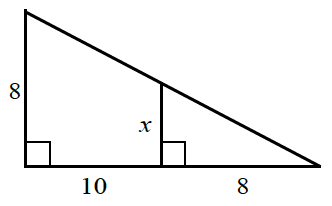### Home > INT1 > Chapter 7 > Lesson 7.1.3 > Problem7-37

7-37.

Examine the diagram at right. The smaller triangle is similar to the larger triangle. Write a proportion and solve for $x$. It will be helpful to draw the two triangles separately on your paper.

Draw two separate triangles. The larger triangle has base $10 + 8 = 18$.

$\frac{x}{8} = \frac{8}{18}$